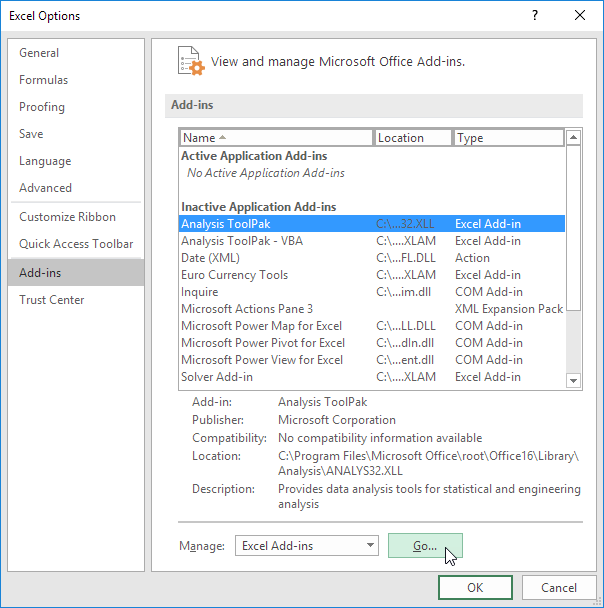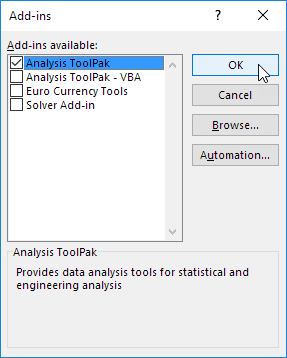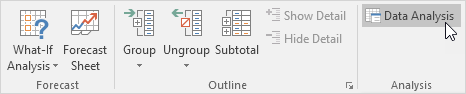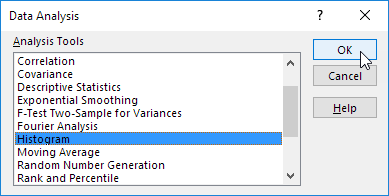## Excel Office

Excel How Tos, Tutorials, Tips & Tricks, Shortcuts

# How To Load Analysis ToolPak in Excel

The Analysis ToolPak is an Excel add-in program that provides data analysis tools for financial, statistical and engineering data analysis.

You provide the data and parameters for each analysis, and the tool uses the appropriate statistical or engineering macro functions to calculate and display the results in an output table. Some tools generate charts in addition to output tables.

Also See:   Understanding Anova in Excel

1. On the File tab, click Options.

2. Under Add-ins, select Analysis ToolPak and click on the Go button.3. Check Analysis ToolPak and click on OK.4. On the Data tab, in the Analysis group, you can now click on Data Analysis.

Also See:   How to perform a t-Test in ExcelThe following dialog box below appears.

5. For example, select Histogram and click OK to create a Histogram in Excel.N/B:  The data analysis functions can be used on only one worksheet at a time. When you perform data analysis on grouped worksheets, results will appear on the first worksheet and empty formatted tables will appear on the remaining worksheets. To perform data analysis on the remainder of the worksheets, recalculate the analysis tool for each worksheet.
Also See:   Excel Frequency Function Example